The results of load combinations are presented in a window with a set of tables where the most interesting values of each scenario are grouped. This window can be resized with the mouse if needed.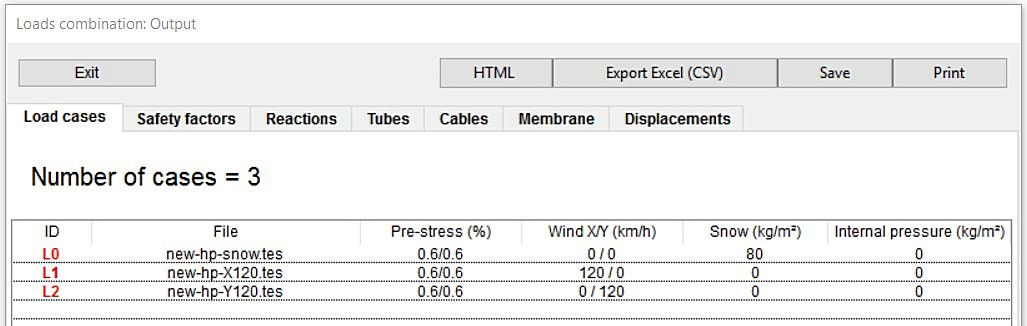In this window, we see a series of buttons whose use is obvious:

• Exit, to leave this window
• Export Excel (CSV), to export the table to a file in CSV format that can be opened with a spreadsheet (e.g. Excel). It doesn’t incorporate format or colors
• Save, to save the data of the table in a text file, TXT format
• Print, to print the table

Below the buttons, we have a strip of 7 tabs, each associated with a table. By default, the first one shows the load cases. In this table we can see:

• The ID of each load case. We can change this value in this table as per our wish.
• The name of the file with the information of the load case.
• The value of the prestress of the membrane
• The value of wind speed (X/Y)
• The value of snow load
• The value of the internal pressure, if it is a pneumatic structure.

Using this table we can give a quick look to the different load cases we are going to combine.

##### Safety Factors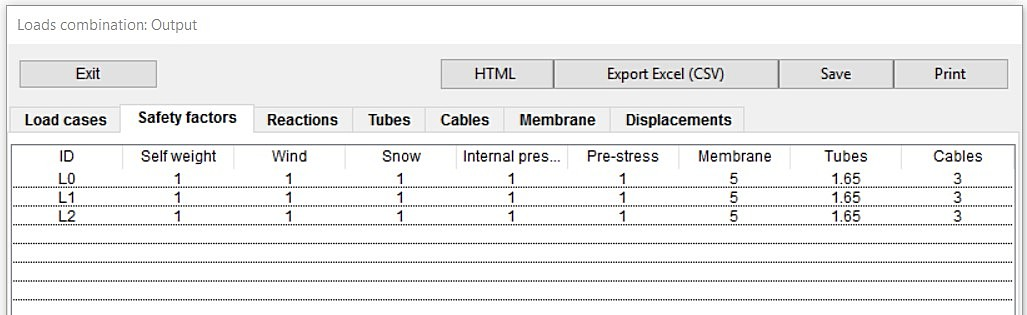In the second tab, we have a table corresponding to safety factors.

Also at a quick glance, we can verify the consistency and correctness of the safety factors on the loads used in the different load cases:

• self weight
• wind
• snow
• internal pressure
• prestress (temperature)

and the safety factors applied to the material, which affect the ratio.

• membrane
• tubes
• cables

##### Reactions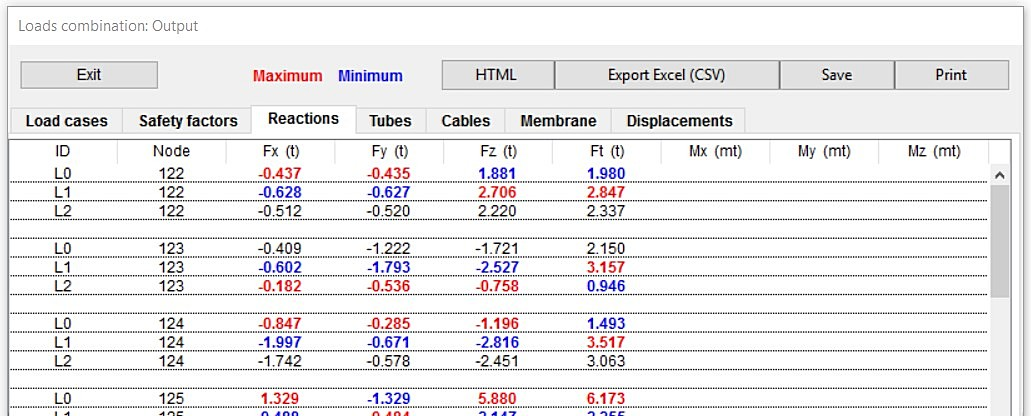In the third tab we have reactions table.

All loads cases and assumptions are grouped responses by each non free node. It shows maximum and minimum reactions in X, Y, Z, total (Ft) for each node and also moments in X, Y, Z if the node belong to a rigid bar.

##### Tubes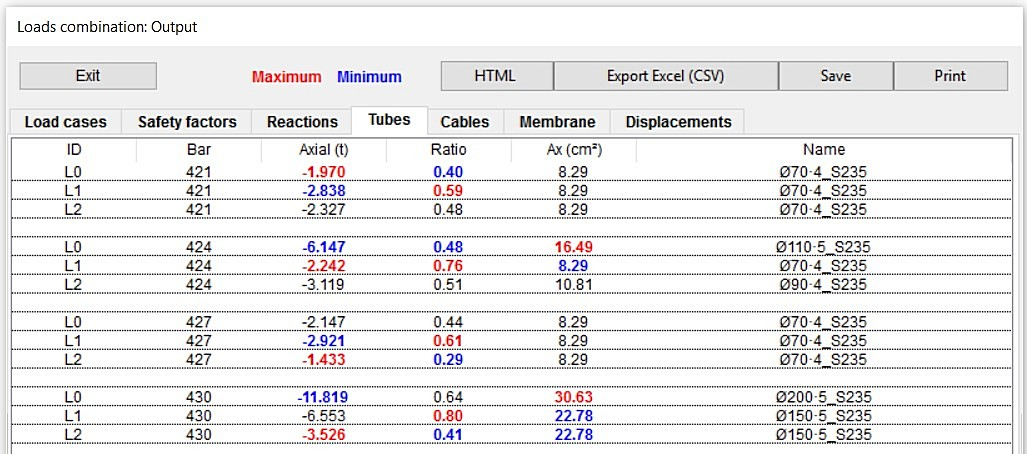On the fourth tab we find the table of tubes.

If the structure has tube type bars, we see the most important values grouped by load cases in this table:

• bar number
• axial force
• ratio of the bar
• cross section area
• description of the tube

In addition to the color criteria (red and blue) in the table as explained above, the Ratio column highlights the values that exceed the limit with yellow, since it shows that something is not working correctly.

##### Cables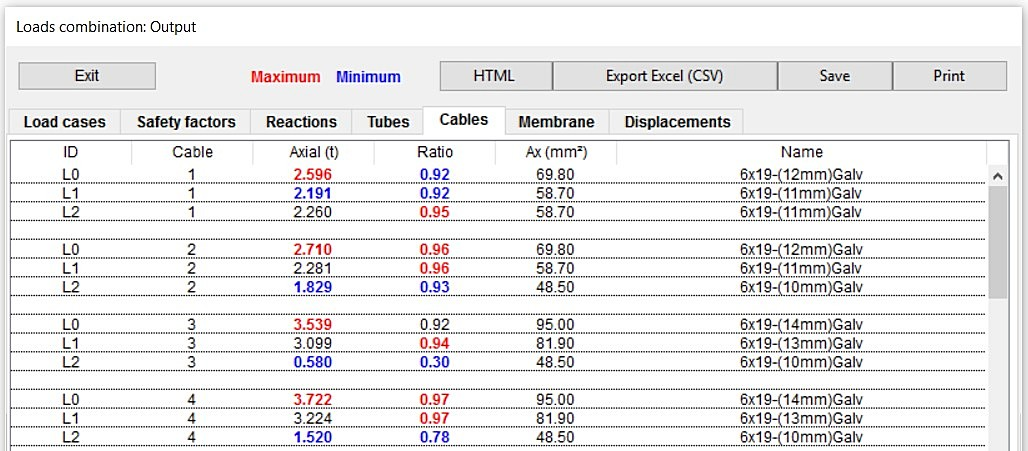On the fifth tab, we find the table of cables.

If the structure has cable type bars, the most important values are grouped by load cases in this table:

• cable number
• axial force
• ratio of the bar
• cross section area
• description of the cable

In addition to the color criteria (red and blue) as in the previous table, the Ratio column highlights the values that exceed the limit with yellow, since it demonstrates that something is not working correctly.

##### Membrane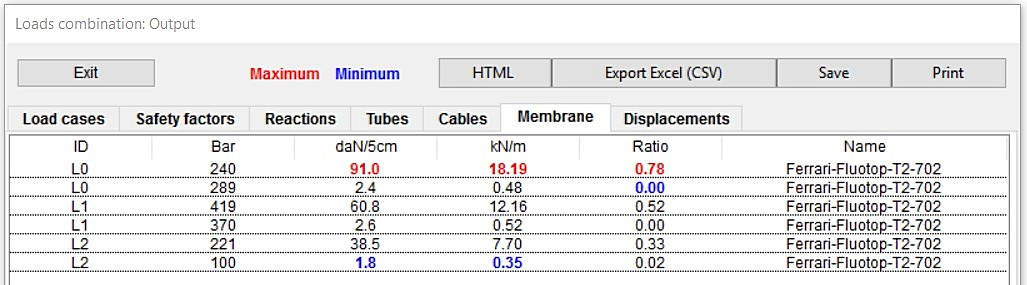In the sixth tab find the table of the membrane.

If the structure has membrane type bars, we see the most important values grouped by load cases in this table :

• Tension in daN/5cm
• tension in kN/m
• ratio of the bar
• description of the membrane

In addition to the color criteria (red and blue) as the other tables, the Ratio column highlights the values that exceed the limit with yellow, since it shows that something is not working correctly.

##### Displacements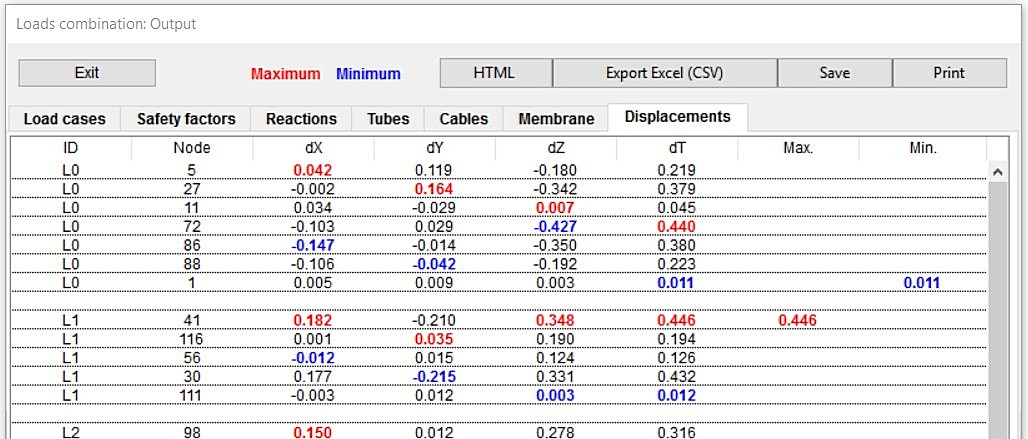In the seventh tab, we find the table of displacement.

It is presents following values for each load case:

• maximum and minimum displacement in X
• maximum and minimum displacement in Y
• maximum and minimum displacement in Z
• maximum and minimum total displacement
• maximum and minimum total displacement of the all load cases

In this table, only free nodes are taken into account, since the fixed ones have null displacement.

The same the color criterion (red and blue) is used as discussed above.